# Henry’s Law – Solutions of gases in gases

The subject of Solutions of gases in gases – Henry’s Law will be discused

## Types of Solutions

– The common solutions that we come across are those where the solute is a solid and the solvent is a liquid.

– In fact, substance in any three states of matter (solid, liquid, gas) can act as solute or solvent.

– Thus there are seven types of solutions whose examples are listed in the following Table.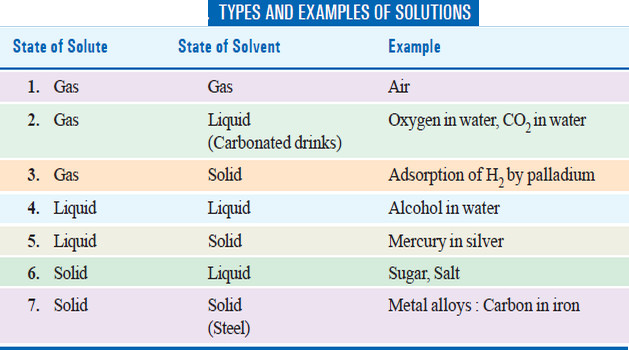– Of the seven types of solutions mentioned in the preivous Table , we will discuss only solutions of gases in gases in detail.

## Solutions of Gases in Gases

– When a gas is mixed with another gas a completely homogeneous solution results provided of course they do not react chemically.

– It is obvious that such gaseous solutions will have the following characteristic properties :

### (1) Complete miscibility

– According to the Kinetic Theory, a gas consists of tiny molecules moving about in vacant space and thus when one gas is dissolved in another gas they form a homogeneous solution quite readily.

– In such a gaseous mixture, the components can be present to an unlimited extent.

### (2) Dalton’s law of Partial pressures

– Since in a gaseous mixture the constituent molecules exist separately, it is obvious that the properties of the mixture will be the sum of properties of the components.

– Thus Dalton (1842) was the first to show that the total pressure exerted by a gaseous mixture is the sum of the individual or partial pressures of the component gases.

– If p1, p2, p3… be the partial pressures of the constituents, the total pressure P of the mixture is given the expression

P = p1 + p2 + p3 + …

– Like other gas laws, Dalton’s law holds strictly only when the partial pressures are not too high.

– This law can be experimentally tested by comparing the total pressure of a gaseous mixture with the sum of the individual pressure of each gas before mixing.

SOLVED PROBLEM

At constant temperature, 250 ml of nitrogen under 720 mm pressure and 380 ml oxygen under 650 mm pressure were put into a one-litre flask. What will be the final pressure of the mixture ?

Solution:

Since PV = P’V’ at constant temperature (Boyle’s Law)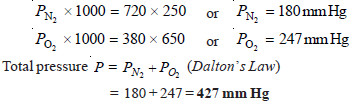## Henry’s Law

– The solubility of a gas in a solvent depends on the pressure and the temperature.

– When a gas is enclosed over its saturated solution, the following equilibrium exists.

gas ↔ gas in solution

– If pressure is increased on the system, the equilibrium will move in the direction which will reduce the pressure (Le Chatelier Principle).

– The pressure can be reduced by more gas dissolving in solvent. Thus solubility or concentration of a gas in a given solvent is increased with increase of pressure.

– A kinetic molecular explanation of the effect of pressure on gas solution system is illustrated in the following Fig.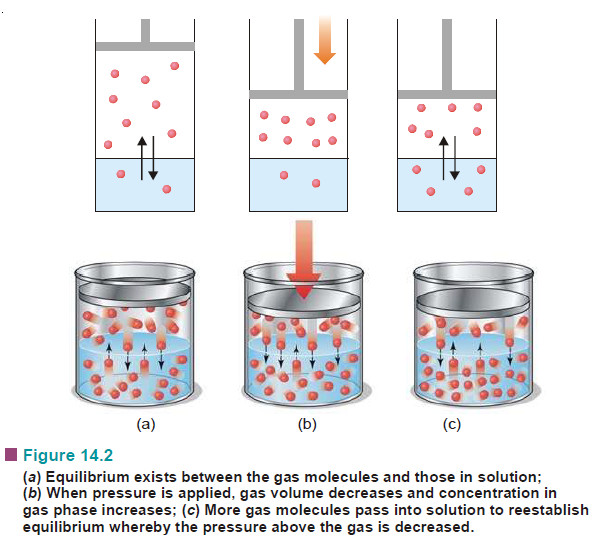– The relationship between pressure and solubility of a gas in a particular solvent was investigated by William Henry (1803).

– He gave a generalisation which is known as Henry’s Law. It may be stated as : for a gas in contact with a solvent at constant temperature, concentration of the gas that dissolves in the solvent is directly proportional to the pressure of the gas.

– Mathematically, Henry’s Law may be expressed as:

C ∝ P

or C = k P

where:

P = pressure of the gas;

C = concentration of the gas in solution;

k = proportionality constant known as Henry’s Law Constant.

The value of (k) depends on the nature of the gas and solvent, and the units of (P) and (C) used.

## Limitations of Henry’s Law

It applies closely to gases with nearly ideal behaviour.

(1) at moderate temperature and pressure.

(2) if the solubility of the gas in the solvent is low.

(3) the gas does not react with the solvent to form a new species. Thus ammonia (or HCl) which react with water do not obey Henry’s Law.

NH3 + H2O ↔ NH4+ + OH

(4) the gas does not associate or dissociate on dissolving in the solvent.

## Solved Problem on Henry’s Law

Proplem (1): The solubility of pure oxygen in water at 20°C and 1.00 atmosphere pressure is 1.38 × 10–3 mole/litre. Calculate the concentration of oxygen at 20°C and partial pressure of 0.21 atmosphere.

Solution:

Calculation of k :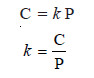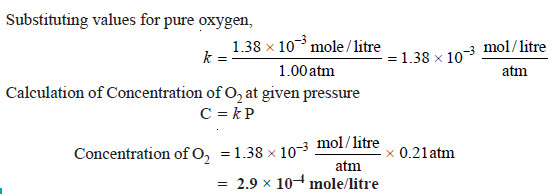Proplem (2): At 20° C the solubility of nitrogen gas in water is 0.0150 g/litre when the partial pressure of N2 is 580 torr. Find the solubility of N2 in H2O at 20°C when its partial pressure is 800 torr.

Solution:

We know that Henry’s law for a gas at two different concentration and pressures can be written as: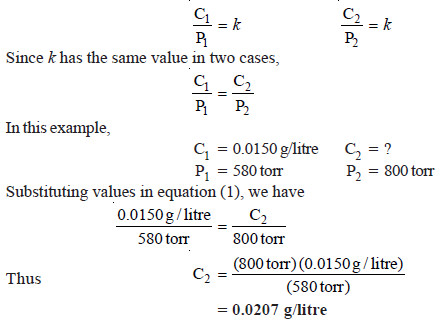Thus solubility of N2 in H2O at 20°C at pressure of 800 torr is 0.0207 g / litre.

Note. The above solution eliminates the calculation of k as was done in example 1.